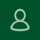It seems that you're using an outdated browser. Some things may not work as they should (or don't work at all).
We suggest you upgrade newer and better browser like: Chrome, Firefox, Internet Explorer or Opera

×
We’ve updated exchange rates and the current ones are as follows:

5.99\$ = 5.49 EUR = 4.89 GBP = 9.19 AUD = 439 RUB = 24.99 PLN = 58 SEK = 59.8 NOK = 40.80 DKK = 5.89 CHF = 8.39 CAD = 34.29 BRL = 43.00 CNY

9.99\$ = 9.19 EUR = 8.19 GBP = 15.29 AUD = 729 RUB = 41.69 PLN = 96.70 SEK = 99.80 NOK = 68.10 DKK = 9.79 CHF = 13.99 CAD = 57.19 BRL = 71.00 CNY

14.99\$ = 13.79 EUR = 12.29 GBP = 22.99 AUD = 1089 RUB = 62.49 PLN = 145.00 SEK = 149.70 NOK = 102.20 DKK = 14.59 CHF = 20.89 CAD = 85.89 BRL = 106.00 CNYJSkull1986: Looks like we have a pretty nice summer sale but I can't seem to buy anything. Is anyone else having this issue? I even tried to add funds to my wallet and it was still a no go.
Please make sure you are not doing the following:

> Purchasing a DLC of which you don't own the basegame on GOG.COM
> Buying a game which you already own

Should none of these apply then please contact us via this form =)
Post edited May 29, 2020 by SmollestLight
high rated
We’ve updated exchange rates and the current ones are as follows:

5.99\$ = 5.09 EUR = 4.59 GBP = 8.29 AUD = 445 RUB = 22.09 PLN = 51.8 SEK = 53 NOK = 37.40 DKK = 5.49 CHF = 7.89 CAD = 32.89 BRL = 41.00 CNY

9.99\$ = 8.39 EUR = 7.59 GBP = 13.89 AUD = 739 RUB = 36.89 PLN = 86.50 SEK = 88.50 NOK = 62.50 DKK = 9.09 CHF = 13.19 CAD = 54.90 BRL = 69.00 CNY

14.99\$ = 12.59 EUR = 11.39 GBP = 20.79 AUD = 1099 RUB = 55.29 PLN = 129.70 SEK = 132.70 NOK = 93.70 DKK = 13.69 CHF = 19.79 CAD = 82.29 BRL = 104.00 CNY
We’ve updated exchange rates and the current ones are as follows:

5.99\$ = 5.19 EUR = 4.69 GBP = 8.49 AUD = 479 RUB = 23.49 PLN = 53.1 SEK = 56.6 NOK = 38.20 DKK = 5.49 CHF = 7.89 CAD = 34.39 BRL = 40.00 CNY

9.99\$ = 8.59 EUR = 7.69 GBP = 14.09 AUD = 799 RUB = 39.09 PLN = 88.60 SEK = 94.40 NOK = 63.60 DKK = 9.19 CHF = 13.19 CAD = 57.29 BRL = 67.00 CNY

14.99\$ = 12.89 EUR = 11.59 GBP = 21.09 AUD = 1199 RUB = 58.59 PLN = 132.90 SEK = 141.70 NOK = 95.50 DKK = 13.79 CHF = 19.79 CAD = 85.99 BRL = 100.00 CNY
high rated
We’ve updated exchange rates and the current ones are as follows:

5.99\$ = 4.99 EUR = 4.49 GBP = 8.09 AUD = 450 RUB = 22.09 PLN = 50.7 SEK = 52.6 NOK = 36.70 DKK = 5.39 CHF = 7.79 CAD = 31.09 BRL = 39.00 CNY

9.99\$ = 8.29 EUR = 7.49 GBP = 13.49 AUD = 749 RUB = 36.89 PLN = 84.50 SEK = 87.80 NOK = 61.30 DKK = 8.99 CHF = 12.89 CAD = 51.79 BRL = 65.00 CNY

14.99\$ = 12.39 EUR = 11.19 GBP = 20.19 AUD = 1129 RUB = 55.29 PLN = 126.80 SEK = 131.70 NOK = 92.00 DKK = 13.39 CHF = 19.39 CAD = 77.79 BRL = 98.00 CNY
high rated
We’ve updated exchange rates and the current ones are as follows:

5.99\$ = 5.09 EUR = 4.39 GBP = 7.79 AUD = 445 RUB = 22.99 PLN = 50.4 SEK = 50.4 NOK = 37.20 DKK = 5.59 CHF = 7.49 CAD = 33.29 BRL = 39.00 CNY

9.99\$ = 8.39 EUR = 7.19 GBP = 12.89 AUD = 739 RUB = 38.29 PLN = 84.60 SEK = 84.10 NOK = 62.10 DKK = 9.29 CHF = 12.49 CAD = 55.51 BRL = 65.00 CNY

14.99\$ = 12.59 EUR = 10.79 GBP = 19.29 AUD = 1099 RUB = 57.39 PLN = 127.00 SEK = 126.20 NOK = 93.2 DKK = 13.99 CHF = 18.69 CAD = 83.19 BRL = 98.00 CNY
high rated
We’ve updated exchange rates and the current ones are as follows:

5.99\$ = 4,99 EUR = 4.29 GBP = 7.79 AUD = 449 RUB = 22.09 PLN = 49.8 SEK = 50.1 NOK = 36.60 DKK = 5.39 CHF = 7.29 CAD = 32.19 BRL = 39.00 CNY

9.99\$ = 8.29 EUR = 7.09 GBP = 12.99 AUD = 739 RUB = 36.89 PLN = 83 SEK = 83.60 NOK = 61 DKK = 8.99 CHF = 12.09 CAD = 53.59 BRL = 64.00 CNY

14.99\$ = 12.39 EUR = 10.59 GBP = 19.39 AUD = 1109 RUB = 55.29 PLN = 124.50 SEK = 125.50 NOK = 91.5 DKK = 13.49 CHF = 18.09 CAD = 80.39 BRL = 96.00 CNY